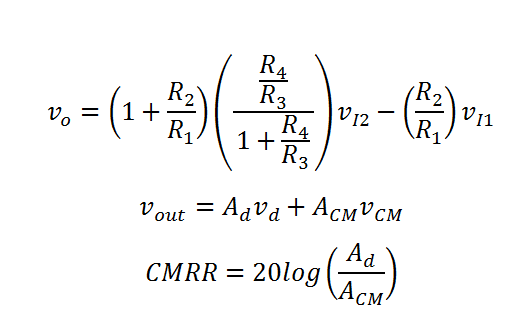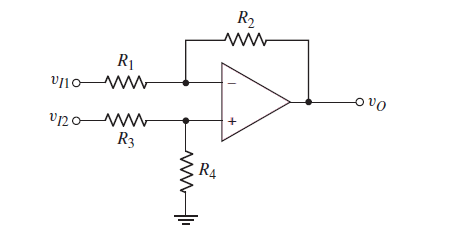# Op-Amp differential Voltage Amplifier and Common Mode Rejection ratio (CMRR) Calculator

This CalcTown calculator calculates the output voltage and common mode rejection ratio of an Op-Amp circuit configuration which amplifies the difference of voltage applied to its two terminals.

K-Ohm
K-Ohm
K-Ohm
K-Ohm
V
V

#### Result

V
dB